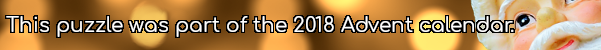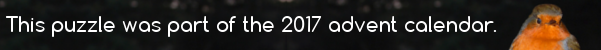mscroggs.co.uk
mscroggs.co.uksubscribe

# Puzzles

## Archive

Show me a random puzzle
▼ show ▼

## 15 DecemberToday's number is smallest three digit palindrome whose digits are all non-zero, and that is not divisible by any of its digits.

## 20 DecemberWhat is the largest number that cannot be written in the form $$10a+27b$$, where $$a$$ and $$b$$ are nonnegative integers (ie $$a$$ and $$b$$ can be 0, 1, 2, 3, ...)?

## Elastic numbers

Throughout this puzzle, expressions like $$AB$$ will represent the digits of a number, not $$A$$ multiplied by $$B$$.
A two-digit number $$AB$$ is called elastic if:
1. $$A$$ and $$B$$ are both non-zero.
2. The numbers $$A0B$$, $$A00B$$, $$A000B$$, ... are all divisible by $$AB$$.
There are three elastic numbers. Can you find them?

## 14 DecemberIn July, I posted the Combining Multiples puzzle.
Today's number is the largest number that cannot be written in the form $$27a+17b$$, where $$a$$ and $$b$$ are positive integers (or 0).

## Combining multiples

In each of these questions, positive integers should be taken to include 0.
1. What is the largest number that cannot be written in the form $$3a+5b$$, where $$a$$ and $$b$$ are positive integers?
2. What is the largest number that cannot be written in the form $$3a+7b$$, where $$a$$ and $$b$$ are positive integers?
3. What is the largest number that cannot be written in the form $$10a+11b$$, where $$a$$ and $$b$$ are positive integers?
4. Given $$n$$ and $$m$$, what is the largest number that cannot be written in the form $$na+mb$$, where $$a$$ and $$b$$ are positive integers?

## Subsum

1) In a set of three integers, will there always be two integers whose sum is even?
2) How many integers must there be in a set so that there will always be three integers in the set whose sum is a multiple of 3?
3) How many integers must there be in a set so that there will always be four integers in the set whose sum is even?
4) How many integers must there be in a set so that there will always be three integers in the set whose sum is even?

## Fill in the digits

Source: Chalkdust
Can you place the digits 1 to 9 in the boxes so that the three digit numbers formed in the top, middle and bottom rows are multiples of 17, 25 and 9 (respectively); and the three digit numbers in the left, middle and right columns are multiples of 11, 16 and 12 (respectively)?

## Always a multiple?

Source: nrich
Take a two digit number. Reverse the digits and add the result to your original number. Your answer is multiple of 11.
Prove that the answer will be a multiple of 11 for any starting number.
Will this work with three digit numbers? Four digit numbers? $$n$$ digit numbers?
© Matthew Scroggs 2019